The process of achieving uniform electrostatic stress in the dielectric of cables is known as grading of cables.

It has already been shown that electrostatic stress in a single core cable has a maximum value (gmax) at the conductor surface and goes on decreasing as we move towards the sheath. The maxi­mum voltage that can be safely applied to a cable depends upon gmax i.e., electrostatic stress at the conductor surface. For safe working of a cable having homogeneous dielectric, the strength of dielectric must be more than gmax. If a dielectric of high strength is used for a cable, it is useful only near the conductor where stress is maximum. But as we move away from the conductor, the electro­static stress decreases, so the dielectric will be unnecessarily overstrong.

The unequal stress distribution in a cable is undesirable for two reasons. Firstly, insulation of greater thickness is required which increases the cable size. Secondly, it may lead to the breakdown of insulation. In order to overcome above disadvantages, it is necessary to have a uniform stress distribution in cables. This can be achieved by distributing the stress in such a way that its value is increased in the outer layers of dielectric. This is known as grading of cables. The following are the two main methods of grading of cables :

The process of achieving uniformity in the dielectric stress by using layers of different dielectrics is known as capacitance grading.

In capacitance grading, the homogeneous dielectric is replaced by a composite dielectric. The composite dielectric consists of various layers of different dielectrics in such a manner that relative permittivity er of any layer is inversely proportional to its distance from the centre. Under such conditions, the value of potential gra­dient at any point in the dieletric is constant and is independent of its distance from the centre. In other words, the dielectric stress in the cable is same everywhere and the grading is ideal one. However, ideal grading requires the use of an infinite number of dielectrics which is an impossible task. In practice, two or three dielectrics are used in the decreasing order of permittivity ; the dielectric of highest permittivity being used near the core.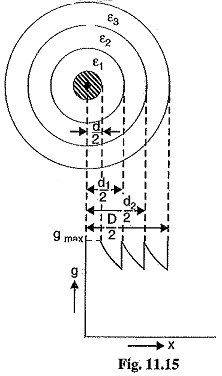The capacitance grading can be explained beautifully by re­telling to Fig. 11.15. There are three dielectrics of outer diameter d1, d2 and D and of relative permittivity ε12 and ε3 respectively. If the permittivities are such that ε> ε> ε3 and the three dielec­trics are worked at the same maximum stress, then,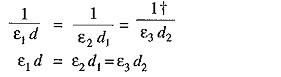Potential difference across the inner layer is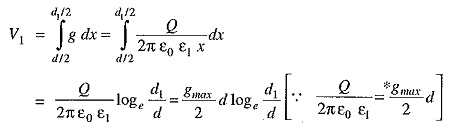Similarly, potential across second layer (V2) and third layer (V3) is given by ;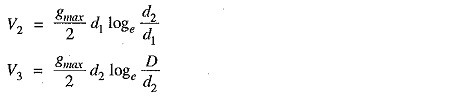Total p.d. between core and earthed sheath is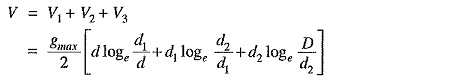If the cable had:homogeneous dielectric then, for the same values of d, D and gmax, the permissible potential difference between core and earthed sheath would have been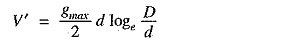Obviously, V > V′ i.e., for given dimensions of the cable, a graded cable can be worked at a greater potential than non-graded cable. Alternatively, for the same safe potential, the size of graded cable will be less than that of non-graded cable. The following points may be noted :

• As the permissible values of gmax are peak values, therefore, all the voltages in above ex­pressions should be taken as peak values and not the r.m.s. values.
• If the maximum stress in the three dielectrics is not the same, then,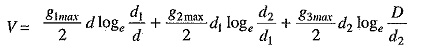The principal disadvantage of this method is that there are a few high grade dielectrics of reason­able cost whose permittivities vary over the required range.

In this method of cable grading, a homogeneous dielectric is used, but it is divided into, various layers by placing metallic intersheaths between the core and lead sheath. The intersheaths are held at suitable potentials which are inbetween the core potential and earth potential. This arrangement improves voltage distribution in the dielectric of the cable and consequently more uniform potential gradient is obtained.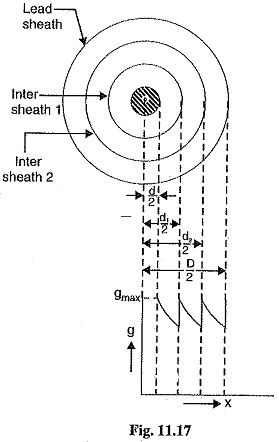Consider a cable of core diameter d and outer lead sheath of diameter D. Suppose that two intersheaths of diameters d1 and d2 are inserted into the homogeneous di­electric and maintained at some fixed potentials. Let V1, V2 and V3 respectively be the voltage between core and intersheath I, between intersheath 1 and 2 and between intersheath 2 and outer lead sheath. As there is a definite potential difference between the inner and outer layers of each intersheath, therefore each sheath can be treated like a homogeneous single core cable.

Maximum stress between core and intersheath 1 is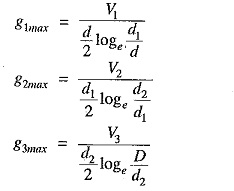Since the dielectric is homogeneous, the maximum stress in each layer is the same i.e.,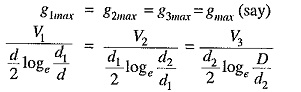As the cable behaves like three capacitors in series, therefore, all the potentials are in phase i.e. Voltage between conductor and earthed lead sheath is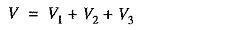Intersheath grading has three principal disadvantages. Firstly, there are complications in fixing the sheath potentials. Secondly, the intersheaths are likely to be damaged during transportation and installation which might result in local concentrations of potential gradient. Thirdly, there are con­siderable losses in the intersheaths due to charging currents. For these reasons, intersheath grading is rarely used.

Scroll to Top